# SOCR EduMaterials AnalysisActivities TwoPairedSign

## SOCR Analysis - Two Paired Sample Sign Test Example

Go to the SOCR Two Paired Sample Sign Test Applet. We will demonstrate Two Paired Sign Test with a SOCR built-in example. This example is based on a dataset from the statistical program "R." For more information of the R program, please see CRAN Home Page. The dataset used here is "shoes" under R's "MASS" library. In the dataset, ten boys are given to the wear of shoes of materials A and B for one foot. We'd like to find if one material is better than the other.

Here's the steps of the activity:

1. Click on Two Paired Sample Sign Test at the left panel's combo box.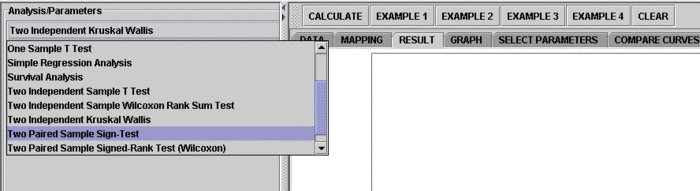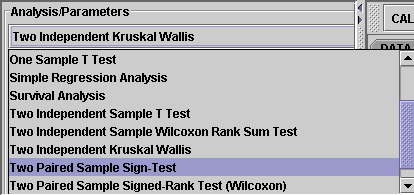On the right panel, first click on EXAMPLE 3 and next click on "Data" to retreive the demonstrated example. This is the example we'll be looking at.Note that the data are difference of B - A.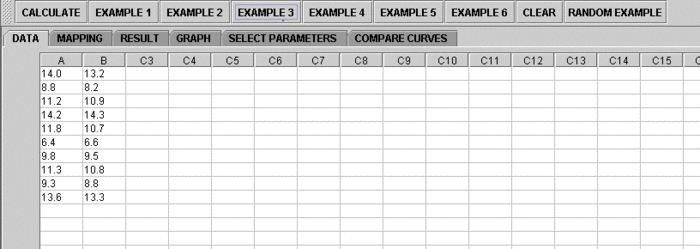2. Click on the "Mapping" button to get to the "Mapping" panel. Click on "ADD" under VARIABLE 1 to add A and click on ADD under VARIABLE 2 to add B. We care about the difference, so which on goes to VARIABLE 1 or VARIABLE 2 is arbitrary. Here, the computer will set difference = Variable 2 - Variable 1. If you like the subtraction to go the other way around, simple assign VARIABLE 1 and VARIABLE 2 in the opposite way.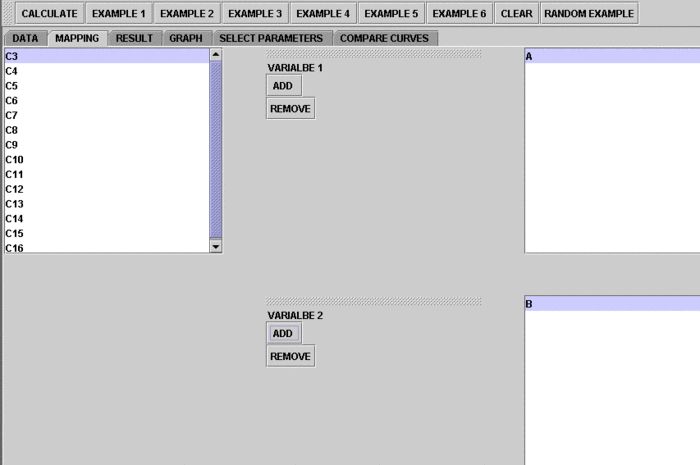3. Click on "Calculate" then "Result" to see the results.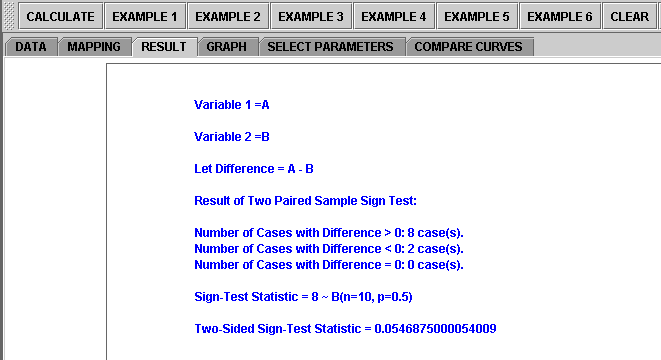Questions for students: By looking at the test results, what do you think about the data? What can you say about the two different shoe materials?